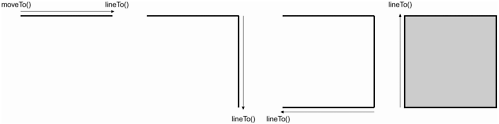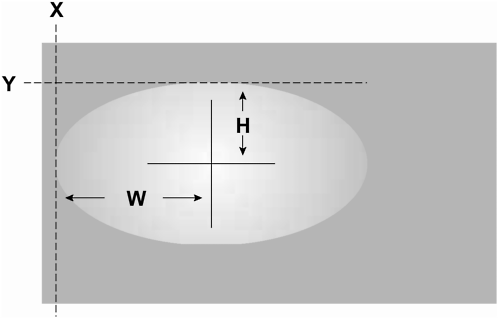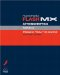# CREATING FILLED SHAPES DYNAMICALLY

 Even though we're not going to devote an exercise to the topic, drawing filled shapes is still worth discussing. By building on the syntax you've used thus far in this lesson, you can use the drawing methods to create a shape and then fill it with a single color (a flat fill) or a gradient (a gradient fill). To create a flat fill, you must let Flash know that the shape about to be drawn will be filled. You do this by employing the following method: ` path.beginFill(color,alpha) ` The path points to the timeline where the lines will exist. The color parameter accepts a hex color value. The second parameter, alpha , accepts a number between 0 and 100 to set the alpha level of the fill. To let Flash know when you're finished drawing a shape, you would use the following method: ` path.endFill() ` Here is an example use of this code: ` _root.createEmptyMovieClip("box",1); with (_root.box) { lineStyle(0,0x000000,100); beginFill(0x990000,100); moveTo(0,0); lineTo(100,0); lineTo(100,100); lineTo(0,100); lineTo(0,0); box.endFill(); } ` The above ActionScript does the following, Creates an empty movie clip instance Sets the line style Initiates the fill Draws a shape Ends the fill It's important to note that when creating a filled shape, the starting point of the shape (as defined by moveTo() ) must also be the ending point of the shape (as defined by the last lineTo() ).Creating a gradient fill is a little more difficult than creating a flat fill; understanding how the gradient fill works is much more difficult. You must first tell Flash that the shape you're about to draw is to be filled with a gradient. The syntax for this is as follows: ` path.beginGradientFill (type, colors, alphas, ratios, matrix) ` The first parameter, type , accepts the string linear or radial. The colors parameter is an array of hex color values that you want to use in your gradient. This array can contain two or more elements. The third parameter, alphas , is an array of alpha values to be applied to each respective color. This array should have the same number of elements as the colors array. The ratios parameter is an array of elements that contain values between 0 and 255. These values determine the color distribution. The matrix parameter of the beginGradientFill() method deserves some attention. This object contains values that are used to move, skew, and rotate the gradient. There are two ways to configure the matrix object the more common of which contains the following properties: matrixType: This is a variable with a value of "box". You must set it so that Flash knows which type of matrix you're using. x: This is the x position at which to start the gradient. Flash uses the upper lefthand corner of the overall gradient to place this gradient. y: This is the y position at which to start the gradient. Flash uses the upper lefthand corner of the overall gradient to place this gradient. w: This is the width of the gradient. h: This is the height of the gradient. r: This is the rotation of the gradient (in radians).Here is an example of the syntax used to create a gradient-filled shape: ` _root.createEmptyMovieClip("holder", 1); with (_root.holder) { lineStyle(0, 0x000000, 0); rotation = 90 * (Math.PI/180); colors = [ 0x6666FF, 0xFF6600 ]; alphas = [ 100, 100 ]; ratios = [ 0, 255 ]; matrix = { matrixType:"box", x:0, y:150, w:200, h:100, r:rotation }; beginGradientFill( "linear", colors, alphas, ratios, matrix ); moveTo(0,0); lineTo(550,0); lineTo(550,300); lineTo(0,300); lineTo(0,0); endFill(); } ` The above ActionScript creates a square and then fills it with a gradient. The lines of the square have an alpha of zero so you cannot see them. You'll probably need to test beginGradientFill() a few times to feel comfortable using it.Macromedia Flash MX ActionScripting: Advanced Training from the Source
ISBN: 0201770229
EAN: 2147483647
Year: 2002
Pages: 161